# Lists in Lisp and Scheme Lists in Lisp

• Slides: 24
Download presentationLists in Lisp and Scheme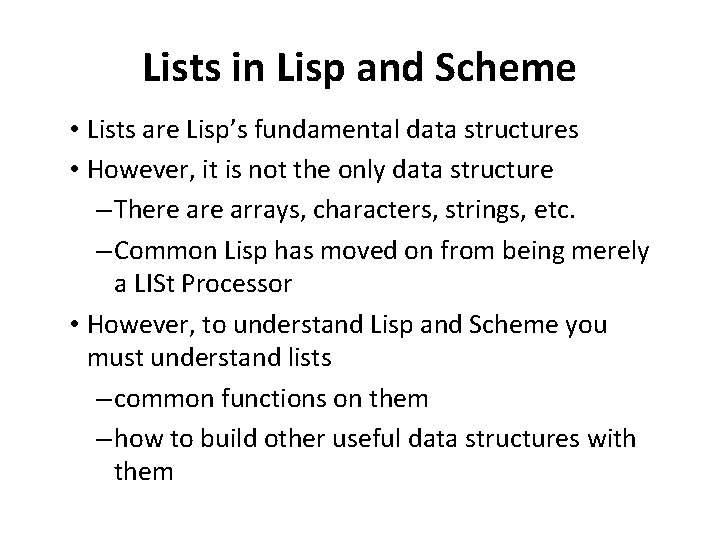Lists in Lisp and Scheme • Lists are Lisp’s fundamental data structures • However, it is not the only data structure – There arrays, characters, strings, etc. – Common Lisp has moved on from being merely a LISt Processor • However, to understand Lisp and Scheme you must understand lists – common functions on them – how to build other useful data structures with them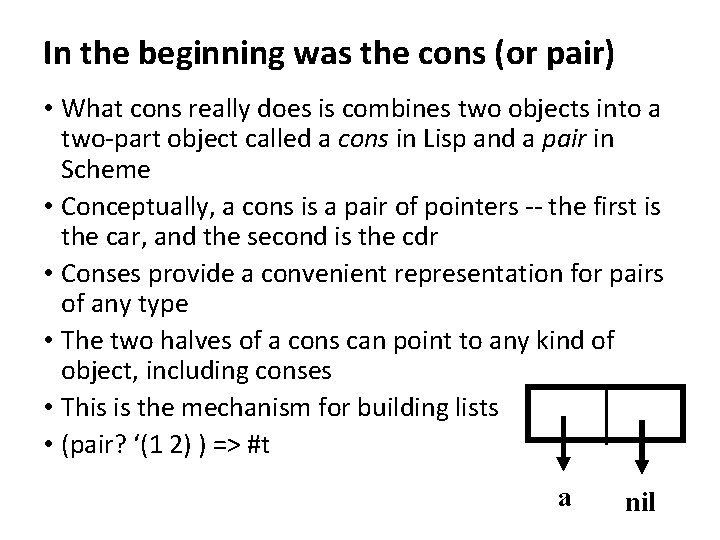In the beginning was the cons (or pair) • What cons really does is combines two objects into a two-part object called a cons in Lisp and a pair in Scheme • Conceptually, a cons is a pair of pointers -- the first is the car, and the second is the cdr • Conses provide a convenient representation for pairs of any type • The two halves of a cons can point to any kind of object, including conses • This is the mechanism for building lists • (pair? ‘(1 2) ) => #t a nil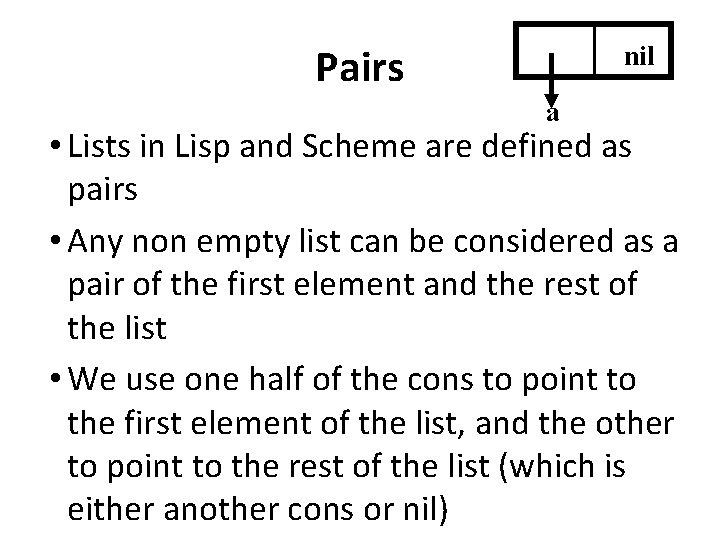Pairs nil a • Lists in Lisp and Scheme are defined as pairs • Any non empty list can be considered as a pair of the first element and the rest of the list • We use one half of the cons to point to the first element of the list, and the other to point to the rest of the list (which is either another cons or nil)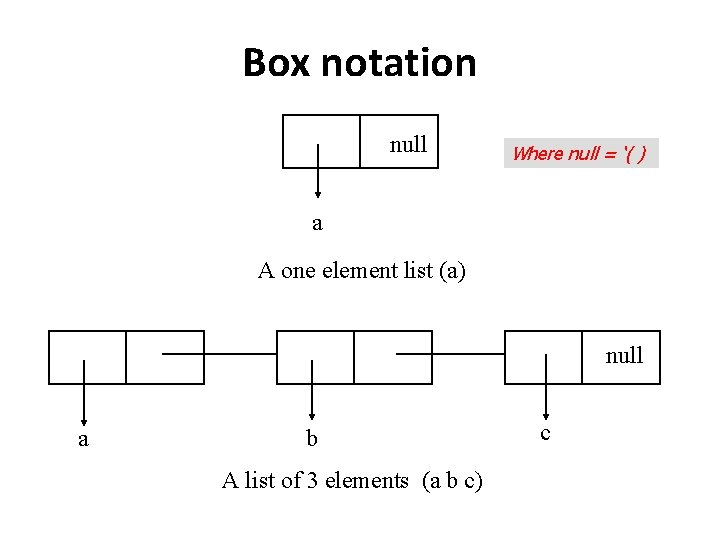Box notation null Where null = ‘( ) a A one element list (a) null a b A list of 3 elements (a b c) c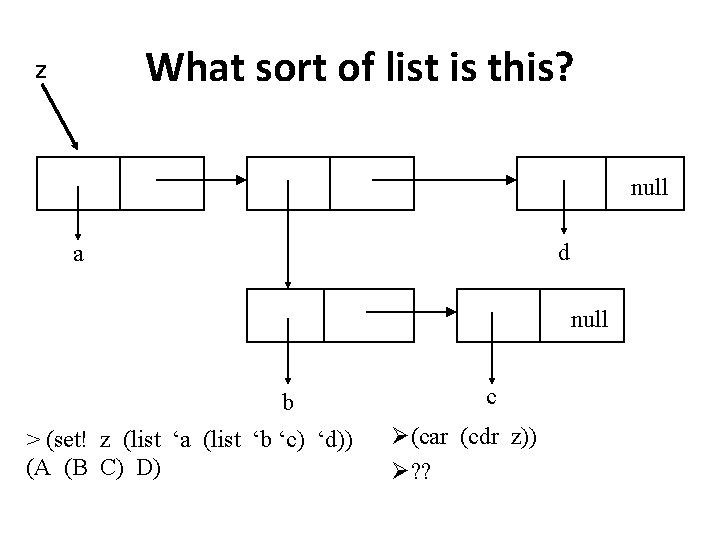What sort of list is this? Z null d a null b > (set! z (list ‘a (list ‘b ‘c) ‘d)) (A (B C) D) c Ø(car (cdr z)) Ø? ?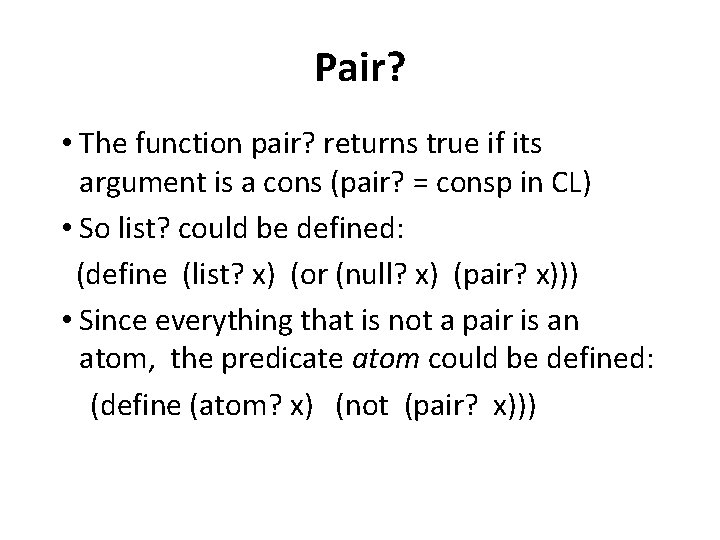Pair? • The function pair? returns true if its argument is a cons (pair? = consp in CL) • So list? could be defined: (define (list? x) (or (null? x) (pair? x))) • Since everything that is not a pair is an atom, the predicate atom could be defined: (define (atom? x) (not (pair? x)))Equality • Each time you call cons, Scheme allocates a new memory with room for two pointers • If we call cons twice with the same arguments, we get back two values that look the same, but are in fact distinct objects: > (define l 1 (cons 'a ‘())) (A) Ø(define l 2 (cons 'a nil))) Ø(A) Ø(eq? l 1 l 2) Ø#f Ø(equal? l 1 l 2) Ø#t Ø(and (eq? (car l 1)(car l 2)) (eq? (cdr l 1)(cdr l 2))) Ø#t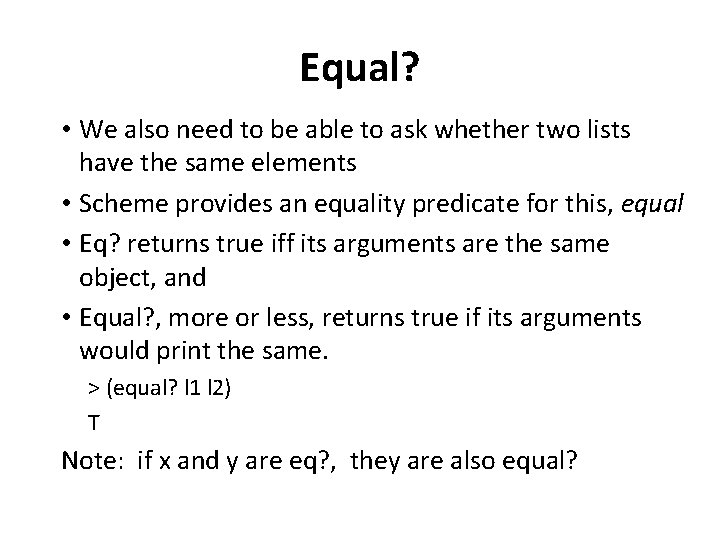Equal? • We also need to be able to ask whether two lists have the same elements • Scheme provides an equality predicate for this, equal • Eq? returns true iff its arguments are the same object, and • Equal? , more or less, returns true if its arguments would print the same. > (equal? l 1 l 2) T Note: if x and y are eq? , they are also equal?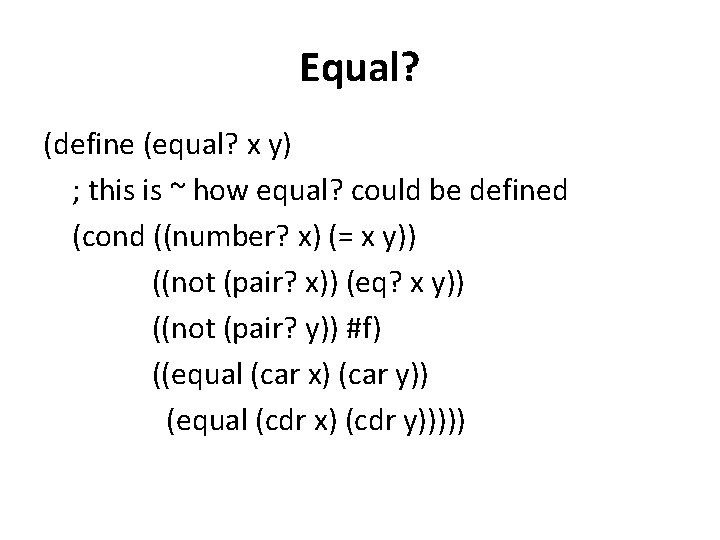Equal? (define (equal? x y) ; this is ~ how equal? could be defined (cond ((number? x) (= x y)) ((not (pair? x)) (eq? x y)) ((not (pair? y)) #f) ((equal (car x) (car y)) (equal (cdr x) (cdr y)))))Does Lisp have pointers? • A secrets to understanding Lisp is to realize that variables have values in the same way that lists have elements • As pairs have pointers to their elements, variables have pointers to their values • What happens, for example, when we set two variables to the same list: > (set! x ‘(a b c)) (A B C) This is just like > (set! y x) in Java (A B C)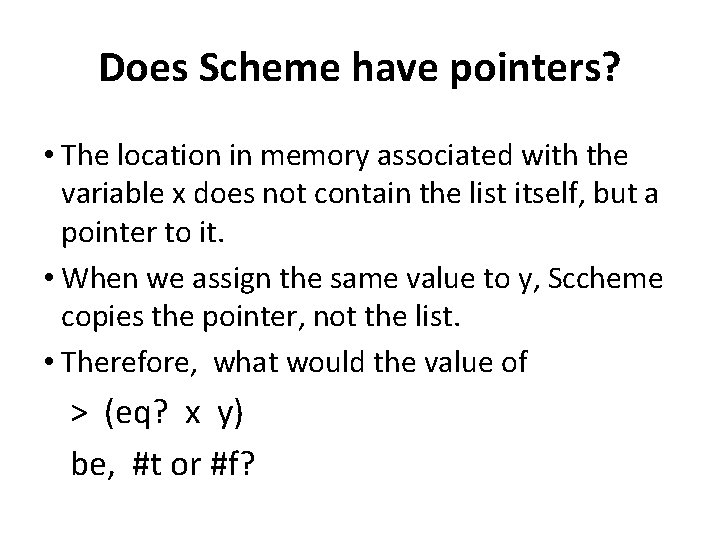Does Scheme have pointers? • The location in memory associated with the variable x does not contain the list itself, but a pointer to it. • When we assign the same value to y, Sccheme copies the pointer, not the list. • Therefore, what would the value of > (eq? x y) be, #t or #f?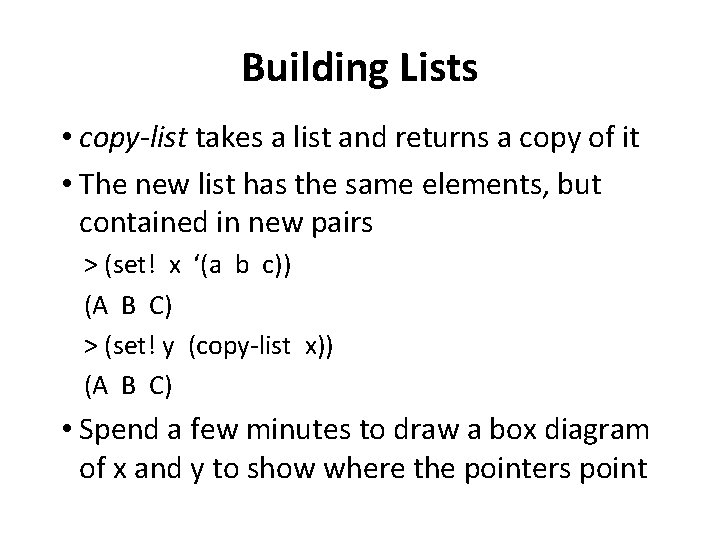Building Lists • copy-list takes a list and returns a copy of it • The new list has the same elements, but contained in new pairs > (set! x ‘(a b c)) (A B C) > (set! y (copy-list x)) (A B C) • Spend a few minutes to draw a box diagram of x and y to show where the pointers point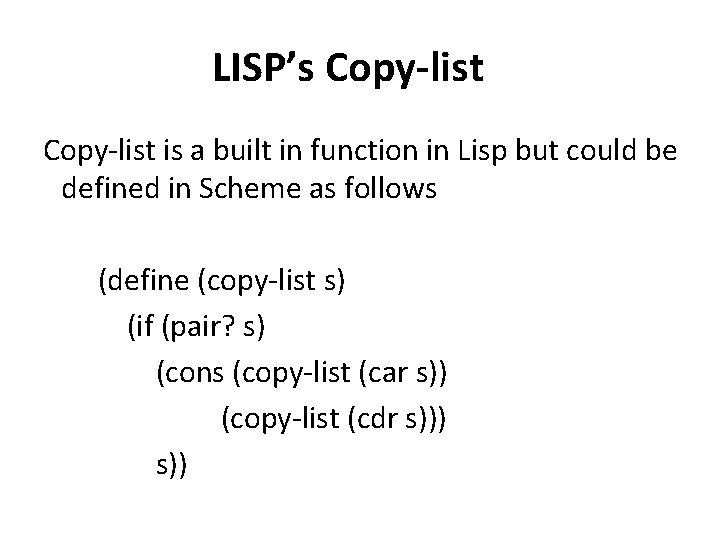LISP’s Copy-list is a built in function in Lisp but could be defined in Scheme as follows (define (copy-list s) (if (pair? s) (cons (copy-list (car s)) (copy-list (cdr s))) s))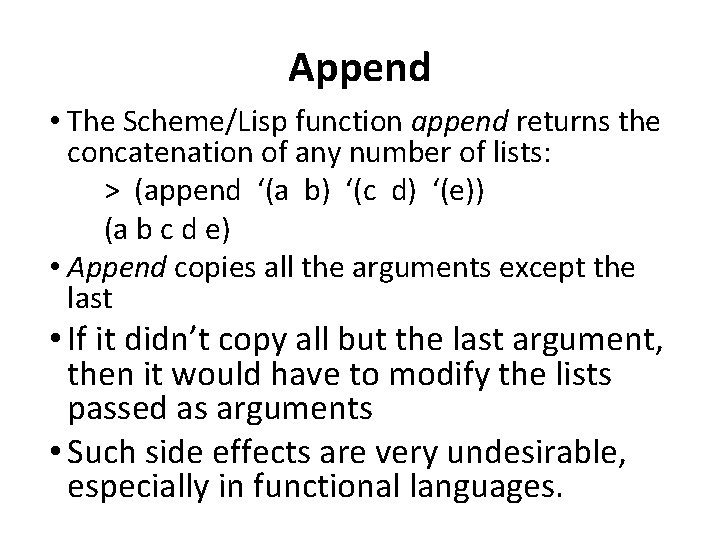Append • The Scheme/Lisp function append returns the concatenation of any number of lists: > (append ‘(a b) ‘(c d) ‘(e)) (a b c d e) • Append copies all the arguments except the last • If it didn’t copy all but the last argument, then it would have to modify the lists passed as arguments • Such side effects are very undesirable, especially in functional languages.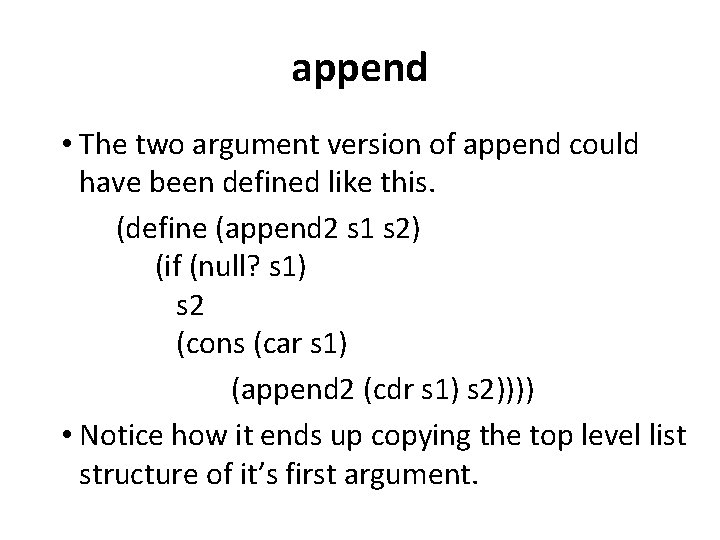append • The two argument version of append could have been defined like this. (define (append 2 s 1 s 2) (if (null? s 1) s 2 (cons (car s 1) (append 2 (cdr s 1) s 2)))) • Notice how it ends up copying the top level list structure of it’s first argument.List access functions • To find the element at a given position in a list use the function list-ref (nth in CL). Ø(list-ref ‘(a b c) 0) Øa • and to find the nth cdr, use list-tail (nthcdr in CL). > (list-tail ‘(a b c) 2) (C) • Both functions are zero indexed.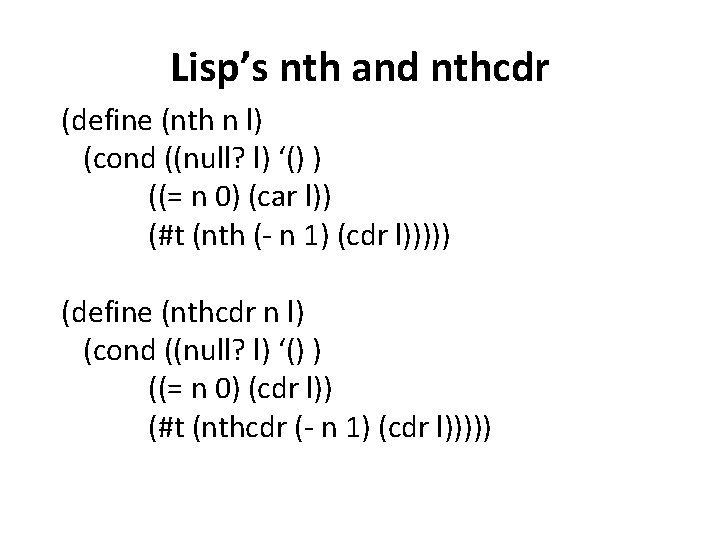Lisp’s nth and nthcdr (define (nth n l) (cond ((null? l) ‘() ) ((= n 0) (car l)) (#t (nth (- n 1) (cdr l))))) (define (nthcdr n l) (cond ((null? l) ‘() ) ((= n 0) (cdr l)) (#t (nthcdr (- n 1) (cdr l)))))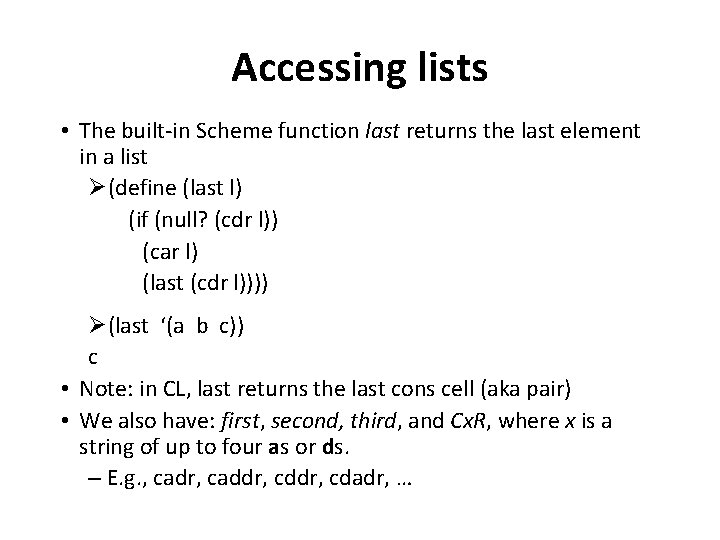Accessing lists • The built-in Scheme function last returns the last element in a list Ø(define (last l) (if (null? (cdr l)) (car l) (last (cdr l)))) Ø(last ‘(a b c)) c • Note: in CL, last returns the last cons cell (aka pair) • We also have: first, second, third, and Cx. R, where x is a string of up to four as or ds. – E. g. , cadr, caddr, cdadr, …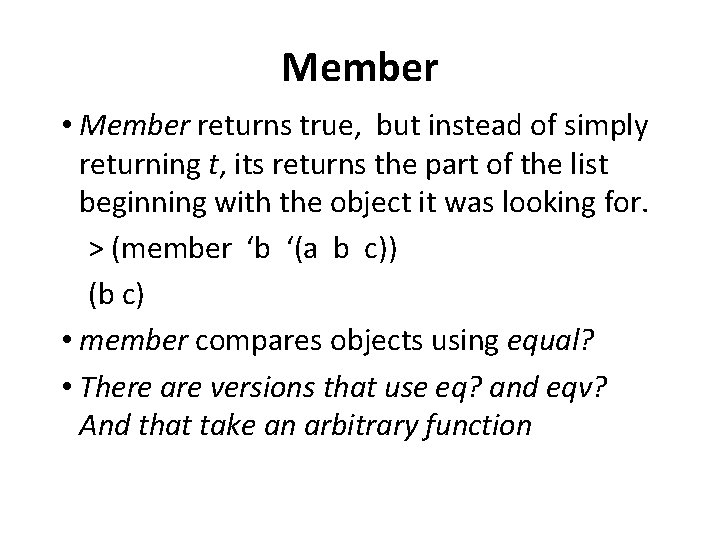Member • Member returns true, but instead of simply returning t, its returns the part of the list beginning with the object it was looking for. > (member ‘b ‘(a b c)) (b c) • member compares objects using equal? • There are versions that use eq? and eqv? And that take an arbitrary function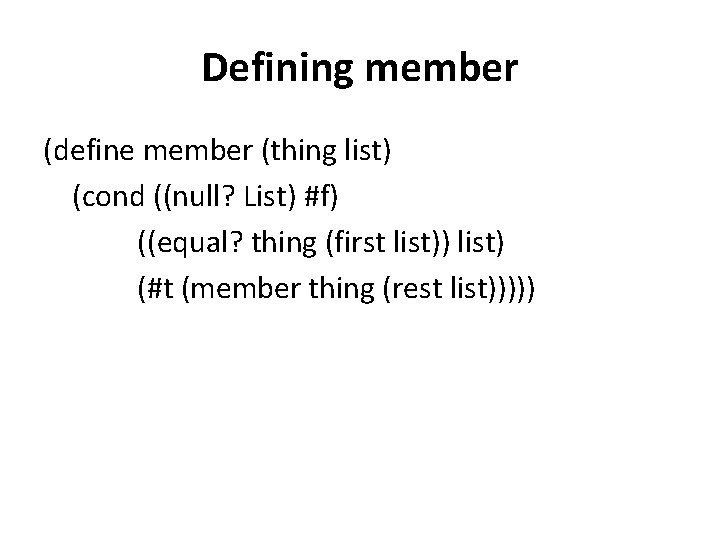Defining member (define member (thing list) (cond ((null? List) #f) ((equal? thing (first list)) list) (#t (member thing (rest list)))))Memf • If we want to find an element satisfying an arbitrary predicate we use the function memf: > (memf odd? ‘(2 3 4)) (3 4) • Which could be defined like: (define (memf f l) (cond ((null? l) #f) ((f (car l)) l) (#t (memf f (cdr l)))))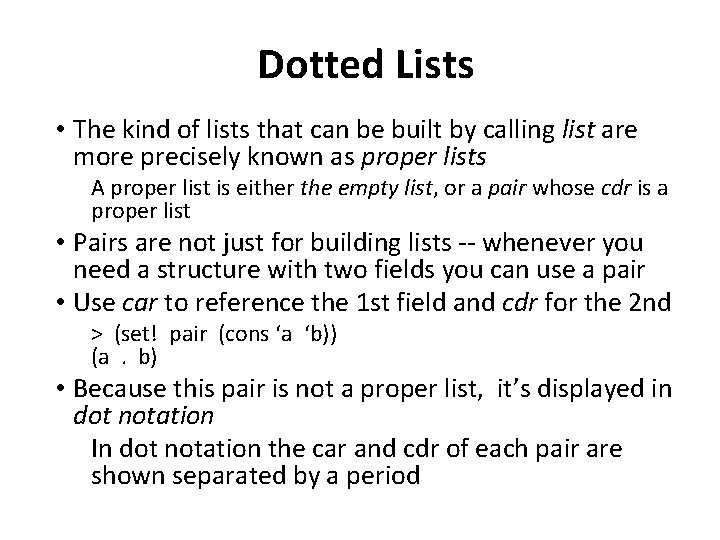Dotted Lists • The kind of lists that can be built by calling list are more precisely known as proper lists A proper list is either the empty list, or a pair whose cdr is a proper list • Pairs are not just for building lists -- whenever you need a structure with two fields you can use a pair • Use car to reference the 1 st field and cdr for the 2 nd > (set! pair (cons ‘a ‘b)) (a. b) • Because this pair is not a proper list, it’s displayed in dot notation In dot notation the car and cdr of each pair are shown separated by a period• A pair that isn’t a proper list is called a dotted pair • However, remember that a dotted pair isn’t really a list at all • It is a just a two part data structure. a b (a. b)